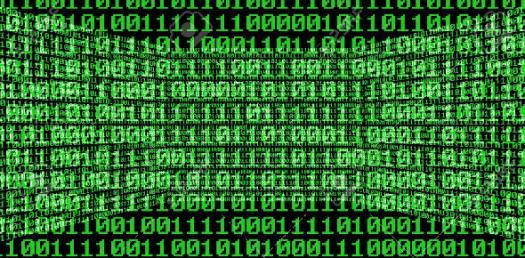# Chapter 1 -assembler Data Representation

15 Questions | Attempts: 226
ShareSettings.

• 1.
Specify the 3 computer trend waves
• A.

1st Wave, 2nd Wave, 3th Wave

• B.

Mechanical Wave, Electric Wave , Electronic Wave

• C.

None all of the Above

• 2.
The smallest unit of data on a binary computer is a single _____
• 3.
The hexadecimal code(BCD) represent _____ different values
• 4.
The byte is considered as the most important data structures used by the 80x86 micropocesor
• A.

True

• B.

False

• 5.
Right shift operation moves each bit in a bits string one psition to the right replacing with 0 high bit and carry out bit 0
• A.

True

• B.

False

• 6.
Main processor that establishes the basic Instruction Set is Intel's x8086
• A.

True

• B.

False

• 7.
Left shift operation moves each bit in a bits string one psition to the left replacing with 0 low bit and carry out bit 7
• A.

True

• B.

False

• 8.
Its considered as a pair of words
• A.

• B.

Double Word

• C.

None all of above

• 9.
Is the smalles addressable datum on the x86 processors
• A.

Byte

• B.

KByte

• C.

A single Bit

• D.

All above are correct

• 10.
Bit ___ is the low order bit or least significant / bit 7 is considered as the   _______ or the highest significant bit of a byte
• 11.
Bit 0..3 compromise the ___________ bits 4..7 form the _______ nibble
• 12.
A group of 16 bits from 0 up to fifteen is considered as
• A.

Double Byte

• B.

16 Bits

• C.

Word

• D.

None all above

• 13.
A collection of bits can be represented by
• A.

Nibbles

• B.

Bytes

• C.

Words and Double Words

• D.

None all of the above

• 14.
A byte can represent 256 numberic values in the range 0..256 and signed numbers in range -____To +_______
• 15.
A binary signed number is considered as positive when H.O is equal to _______ and negative when H.O is equal to ____ type your answer as a number

## Related TopicsBack to top
×

Wait!
Here's an interesting quiz for you.